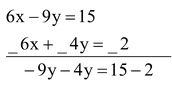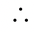# Solution Of Linear Equation In Two Variable

## BY ELIMINATION OF MAKING EQUAL COEFFICIENT:

question . Solve the following equations

2x – 3y = 5

3x + 2y = 1

Solution: Given eq. are 2x – 3y = 5 .....(i)

3x + 2y = 1 ....(ii)

Multiplying 1 eg. (i) by 3 and eg. (ii) by 2 we get

On subtraction⇒ –13y = 13

⇒ y = 13/-13

⇒ y = -1

Put the value of y in eg. (i) we get

2x – (3) × (–1) = 5

2x + 3 = 5

⇒ 2x = 5 - 3

⇒ 2x = 2

⇒ x = 1x = 1, y = 1 Ans.

SUBSTITUTION METHOD:

question . Solve x + 4y = 14 ....(i)

7x – 3y = 5 ...(ii)

Solution: From equation (i) x = 14 - 4y

Substitute the value of x in equation (ii)

⇒ 7 (14 - 4y) - 3y = 5

⇒ 98 - 28y - 3y = 5

⇒ 98 - 31y = 5

⇒ 93 = 31y

⇒ y = 93/31

⇒ y = 3

Now substitute value of y in equation (ii)

⇒ 7x - 3 (3) = 5

⇒ 7x - 3 (3) = 5

⇒ 7x = 14

⇒ x =  14/7= 2

So, solution is x = 2 and y = 3. Ans.